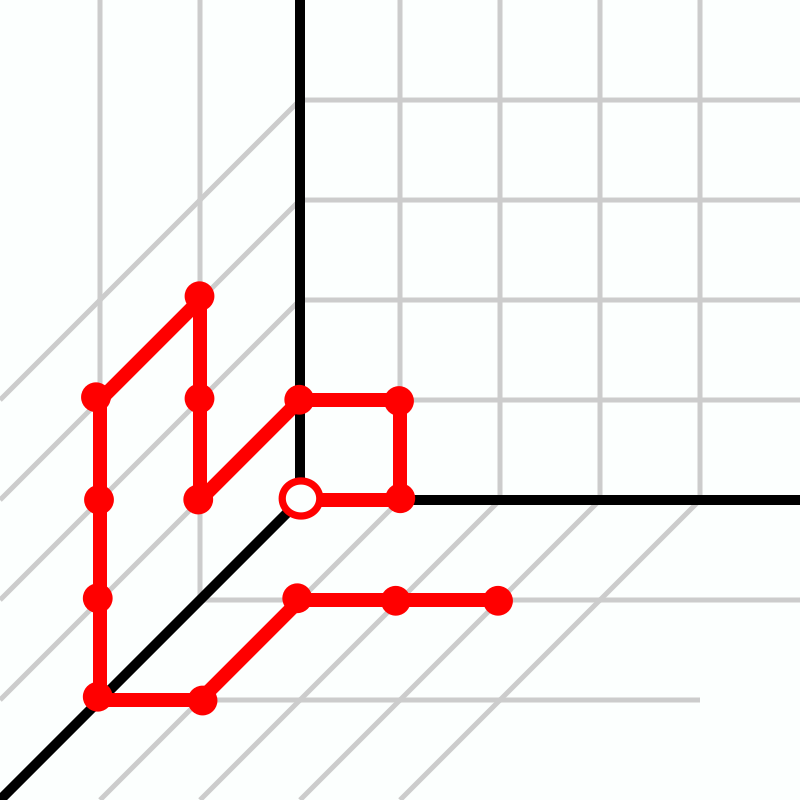## Integer sequences published on the OEIS• A261834 Number of n-step adjacent expansions on the hexagonal lattice.
• A272265 Number of n-step tri-directional self-avoiding walks on the hexagonal lattice.
• A272763 Number of n-step self-avoiding walks on the square lattice with diagonals allowed (Moore neighborhood).
• A330079 Number of n-step self-avoiding walks on first octant of the cubic lattice.
• A283106 Number of distinct envelope areas of the polyominoes of order n.
• A325401 minflip(n) = min(n, r(n)) where r(n) is the binary reverse of n.
• A325402 maxflip(n) = max(n, r(n)) where r(n) is the binary reverse of n.
• A322142 Powernacci numbers: a(n) = 2^(a(n-1) + a(n-2)) with a(0) = 0 and a(1) = 1.